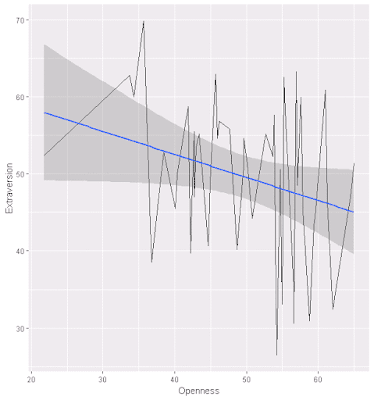### Naive Bayes on Political Outcome Based on State-level Big Five Assessment

As part of another Pluralsight training presentation, Data Mining Algorithms in SSAS, Excel, and R, I worked through various exercises, and from that I've adapated Naive Basyes to one of my existing data sets.

The code is below, as are some related graphs. Overall, the percent correct predicted based on Big Five personality traits using the Naive Bayes calculation is 66%.

Source data is here.

``````
#
#

# review data
nrow(BigFivByState.df)
summary(BigFivByState.df)
names(BigFivByState.df)

# various aggregations
# as "count this value" ~ grouped by this + this
Liberal.dist <- aggregate(State ~ Liberal, data = BigFivByState.df, FUN = length)

RedBlue.dist <- aggregate(State ~ Politics, data = BigFivByState.df, FUN = length)

#
# plotting
#

# install ggplot
install.packages('ggplot2')
library(ggplot2)

# simple count per group
Political.dist <- ggplot(BigFivByState.df, aes(Politics), FUN = length)
Political.dist + geom_bar()# charts with trendlines
cor.test(BigFivByState.df\$Conscientiousness, BigFivByState.df\$Openness)
OpennessVersusConscientiousness <- ggplot(BigFivByState.df, aes(x = Conscientiousness, y = Openness), FUN = length, na.rm = TRUE)
OpennessVersusConscientiousness + geom_line(na.rm = TRUE) + geom_smooth(method = lm, na.rm = TRUE)

cor.test(BigFivByState.df\$Neuroticism, BigFivByState.df\$Openness)
OpennessVersusNeuroticism <- ggplot(BigFivByState.df, aes(x = Neuroticism, y = Openness), FUN = length, na.rm = TRUE)
OpennessVersusNeuroticism + geom_line(na.rm = TRUE) + geom_smooth(method = lm, na.rm = TRUE)

cor.test(BigFivByState.df\$Extraversion, BigFivByState.df\$Neuroticism)
ExtraversionVersusNeuroticism <- ggplot(BigFivByState.df, aes(x = Neuroticism, y = Extraversion), FUN = length, na.rm = TRUE)
ExtraversionVersusNeuroticism + geom_line(na.rm = TRUE) + geom_smooth(method = lm, na.rm = TRUE)

cor.test(BigFivByState.df\$Extraversion, BigFivByState.df\$Openness)
ExtraversionVersusOpenness <- ggplot(BigFivByState.df, aes(x = Openness, y = Extraversion), FUN = length, na.rm = TRUE)
ExtraversionVersusOpenness + geom_line(na.rm = TRUE) + geom_smooth(method = lm, na.rm = TRUE)#
# Naive Bayes
#

install.packages('e1071', dependencies = TRUE)
library(e1071)

# naive bayes
NaiveBayes <- naiveBayes(BigFivByState.df[, 2:11], BigFivByState.df\$Politics)

# a priori probabilities for the target variables
NaiveBayes\$apriori

# a priori probabilities for the input variables
NaiveBayes\$tables

# data frame with predictions
predictions.df <- as.data.frame(predict(NaiveBayes, BigFivByState.df, type = 'raw'))

#
# Validate Results
#

# join predictions with source
predictions.joined <- cbind(BigFivByState.df, predictions.df)

# validate predictions
predictions.joined\$Predicted <- ifelse(predictions.joined\$Blue >= .05, "Blue", "Red")
predictions.joined\$Check <- ifelse(predictions.joined\$Politics == predictions.joined\$Predicted, "Correct", "Incorrect")

# calculate right and wrong
length(predictions.joined\$Check[predictions.joined\$Check== "Correct"]) / length(predictions.joined\$Check)

# chart count of right and wrong
ggplot(predictions.joined, aes(Check), FUN = length) + geom_bar()# output
write.csv(predictions.joined, file = "JoinedPoliticaLPredictions.csv")

``````